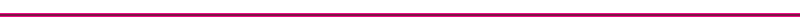Heat Transfer Resistance Modeling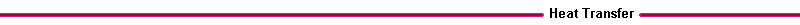Introduction The conduction and convection heat transfer in engines are processes that occur in series and parallel with each other. A series path is convection through the cylinder gas boundary layer, conduction across the cylinder wall, and convection through the coolant liquid boundary layer; and a parallel path is conduction through the cylinder wall and through the piston crown. In heat transfer resistance modeling, we look for regions which have relatively large temperature differences, and compute the heat transfer resistance across those regions.Figure 12. Resistance Network Diagram The thermal resistance is defined as the ratio of the temperature difference, dT, to the heat transfer Q. This is analogous to Ohm's law, in which the electrical resistance is defined as the ratio of the voltage drop across a resistor to the current flow across the resistor. DV = I R or R = DV/ I (Ohm's Law) DT = (Q/A) R or R = DT / (Q/A) (on a per unit area basis) Conduction resistance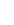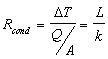Convection resistance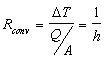The resistance model is very useful in determining the heat transfer in a complex steady state heat transfer situation. It is assumes that the heat transfer is primarily one dimensional across the resistance element, so as the problem becomes more multidimensional, the accuracy decreases. Heat transfer to coolant For the heat transfer from the engine cylinder to the coolant, a series path can be assumed. For example: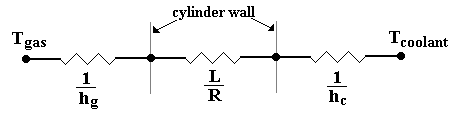Figure 13. Three Resistor Network for Piston Cylinder Wall For example, assume that the cylinder gas temperature is 1200 K, and the coolant temperature is 300 K. The cylinder thermal conductivity is 80 W/mK, and its thickness is ½" ( 0.012 m). Also assume that the convection coefficient is 200 on the gas side, and 1000 on the coolant side. Then The thermal resistance of the gas layer, Rgas, is 1/h = 1/200 = 50 x 10-4 The thermal resistance of the cylinder wall, Rwall is L/k = 0.012/80 = 1.5 x 10-4 The thermal resistance of the coolant, Rcoolant is 1/h = 1/1000 = 10 x 10-4 The largest resistance is the gas side resistance, Rgas . This means that the heat transfer in this case is relatively insensitive to the type of material used in the wall. If the cylinder was made of aluminum instead of steel, the overall heat transfer would not change significantly. For the above resistances, the overall heat transfer is about 150,000 W/m2.